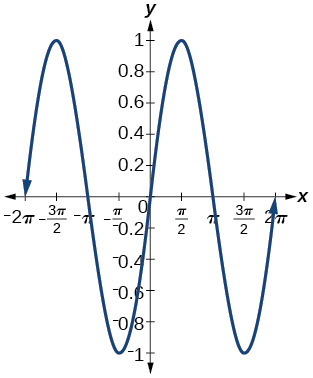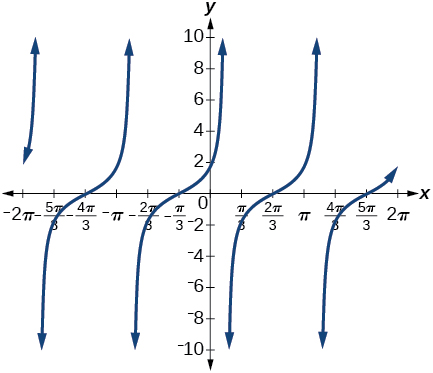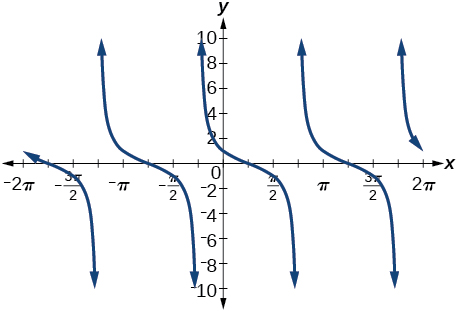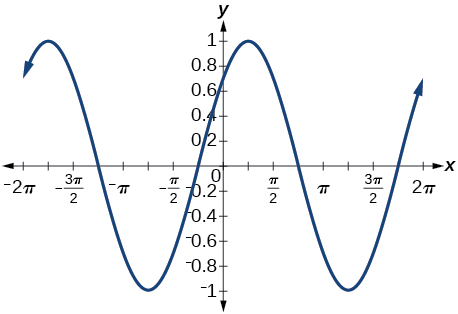# 9.2 Sum and difference identities  (Page 6/6)

 Page 6 / 6

## Verbal

Explain the basis for the cofunction identities and when they apply.

The cofunction identities apply to complementary angles. Viewing the two acute angles of a right triangle, if one of those angles measures $\text{\hspace{0.17em}}x,$ the second angle measures $\text{\hspace{0.17em}}\frac{\pi }{2}-x.\text{\hspace{0.17em}}$ Then $\text{\hspace{0.17em}}\mathrm{sin}x=\mathrm{cos}\left(\frac{\pi }{2}-x\right).\text{\hspace{0.17em}}$ The same holds for the other cofunction identities. The key is that the angles are complementary.

Is there only one way to evaluate $\text{\hspace{0.17em}}\mathrm{cos}\left(\frac{5\pi }{4}\right)?\text{\hspace{0.17em}}$ Explain how to set up the solution in two different ways, and then compute to make sure they give the same answer.

Explain to someone who has forgotten the even-odd properties of sinusoidal functions how the addition and subtraction formulas can determine this characteristic for $\text{\hspace{0.17em}}f\left(x\right)=\mathrm{sin}\left(x\right)\text{\hspace{0.17em}}$ and $\text{\hspace{0.17em}}g\left(x\right)=\mathrm{cos}\left(x\right).\text{\hspace{0.17em}}$ (Hint: $\text{\hspace{0.17em}}0-x=-x$ )

$\mathrm{sin}\left(-x\right)=-\mathrm{sin}x,$ so $\text{\hspace{0.17em}}\mathrm{sin}x\text{\hspace{0.17em}}$ is odd. $\text{\hspace{0.17em}}\mathrm{cos}\left(-x\right)=\mathrm{cos}\left(0-x\right)=\mathrm{cos}x,$ so $\text{\hspace{0.17em}}\mathrm{cos}x\text{\hspace{0.17em}}$ is even.

## Algebraic

For the following exercises, find the exact value.

$\mathrm{cos}\left(\frac{7\pi }{12}\right)$

$\mathrm{cos}\left(\frac{\pi }{12}\right)$

$\frac{\sqrt{2}+\sqrt{6}}{4}$

$\mathrm{sin}\left(\frac{5\pi }{12}\right)$

$\mathrm{sin}\left(\frac{11\pi }{12}\right)$

$\frac{\sqrt{6}-\sqrt{2}}{4}$

$\mathrm{tan}\left(-\frac{\pi }{12}\right)$

$\mathrm{tan}\left(\frac{19\pi }{12}\right)$

$-2-\sqrt{3}$

For the following exercises, rewrite in terms of $\text{\hspace{0.17em}}\mathrm{sin}\text{\hspace{0.17em}}x\text{\hspace{0.17em}}$ and $\text{\hspace{0.17em}}\mathrm{cos}\text{\hspace{0.17em}}x.$

$\mathrm{sin}\left(x+\frac{11\pi }{6}\right)$

$\mathrm{sin}\left(x-\frac{3\pi }{4}\right)$

$-\frac{\sqrt{2}}{2}\mathrm{sin}x-\frac{\sqrt{2}}{2}\mathrm{cos}x$

$\mathrm{cos}\left(x-\frac{5\pi }{6}\right)$

$\mathrm{cos}\left(x+\frac{2\pi }{3}\right)$

$-\frac{1}{2}\mathrm{cos}x-\frac{\sqrt{3}}{2}\mathrm{sin}x$

For the following exercises, simplify the given expression.

$\mathrm{csc}\left(\frac{\pi }{2}-t\right)$

$\mathrm{sec}\left(\frac{\pi }{2}-\theta \right)$

$\mathrm{csc}\theta$

$\mathrm{cot}\left(\frac{\pi }{2}-x\right)$

$\mathrm{tan}\left(\frac{\pi }{2}-x\right)$

$\mathrm{cot}x$

$\mathrm{sin}\left(2x\right)\text{\hspace{0.17em}}\mathrm{cos}\left(5x\right)-\mathrm{sin}\left(5x\right)\text{\hspace{0.17em}}\mathrm{cos}\left(2x\right)$

$\frac{\mathrm{tan}\left(\frac{3}{2}x\right)-\mathrm{tan}\left(\frac{7}{5}x\right)}{1+\mathrm{tan}\left(\frac{3}{2}x\right)\mathrm{tan}\left(\frac{7}{5}x\right)}$

$\mathrm{tan}\left(\frac{x}{10}\right)$

For the following exercises, find the requested information.

Given that $\text{\hspace{0.17em}}\mathrm{sin}\text{\hspace{0.17em}}a=\frac{2}{3}\text{\hspace{0.17em}}$ and $\text{\hspace{0.17em}}\mathrm{cos}\text{\hspace{0.17em}}b=-\frac{1}{4},$ with $\text{\hspace{0.17em}}a\text{\hspace{0.17em}}$ and $\text{\hspace{0.17em}}b\text{\hspace{0.17em}}$ both in the interval $\text{\hspace{0.17em}}\left[\frac{\pi }{2},\pi \right),$ find $\text{\hspace{0.17em}}\mathrm{sin}\left(a+b\right)\text{\hspace{0.17em}}$ and $\text{\hspace{0.17em}}\mathrm{cos}\left(a-b\right).$

Given that $\text{\hspace{0.17em}}\mathrm{sin}\text{\hspace{0.17em}}a=\frac{4}{5},$ and $\text{\hspace{0.17em}}\mathrm{cos}\text{\hspace{0.17em}}b=\frac{1}{3},$ with $\text{\hspace{0.17em}}a\text{\hspace{0.17em}}$ and $\text{\hspace{0.17em}}b\text{\hspace{0.17em}}$ both in the interval $\text{\hspace{0.17em}}\left[0,\frac{\pi }{2}\right),$ find $\text{\hspace{0.17em}}\mathrm{sin}\left(a-b\right)\text{\hspace{0.17em}}$ and $\text{\hspace{0.17em}}\mathrm{cos}\left(a+b\right).$

$\begin{array}{ccccc}\hfill \mathrm{sin}\left(a-b\right)& =& \left(\frac{4}{5}\right)\left(\frac{1}{3}\right)-\left(\frac{3}{5}\right)\left(\frac{2\sqrt{2}}{3}\right)\hfill & =& \frac{4-6\sqrt{2}}{15}\hfill \\ \hfill \mathrm{cos}\left(a+b\right)& =& \left(\frac{3}{5}\right)\left(\frac{1}{3}\right)-\left(\frac{4}{5}\right)\left(\frac{2\sqrt{2}}{3}\right)\hfill & =& \frac{3-8\sqrt{2}}{15}\hfill \end{array}$

For the following exercises, find the exact value of each expression.

$\mathrm{sin}\left({\mathrm{cos}}^{-1}\left(0\right)-{\mathrm{cos}}^{-1}\left(\frac{1}{2}\right)\right)$

$\mathrm{cos}\left({\mathrm{cos}}^{-1}\left(\frac{\sqrt{2}}{2}\right)+{\mathrm{sin}}^{-1}\left(\frac{\sqrt{3}}{2}\right)\right)$

$\frac{\sqrt{2}-\sqrt{6}}{4}$

$\mathrm{tan}\left({\mathrm{sin}}^{-1}\left(\frac{1}{2}\right)-{\mathrm{cos}}^{-1}\left(\frac{1}{2}\right)\right)$

## Graphical

For the following exercises, simplify the expression, and then graph both expressions as functions to verify the graphs are identical. Confirm your answer using a graphing calculator.

$\mathrm{cos}\left(\frac{\pi }{2}-x\right)$

$\mathrm{sin}x$$\mathrm{sin}\left(\pi -x\right)$

$\mathrm{tan}\left(\frac{\pi }{3}+x\right)$

$\mathrm{cot}\left(\frac{\pi }{6}-x\right)$$\mathrm{sin}\left(\frac{\pi }{3}+x\right)$

$\mathrm{tan}\left(\frac{\pi }{4}-x\right)$

$\mathrm{cot}\left(\frac{\pi }{4}+x\right)$$\mathrm{cos}\left(\frac{7\pi }{6}+x\right)$

$\mathrm{sin}\left(\frac{\pi }{4}+x\right)$

$\frac{\mathrm{sin}x}{\sqrt{2}}+\frac{\mathrm{cos}x}{\sqrt{2}}$$\mathrm{cos}\left(\frac{5\pi }{4}+x\right)$

For the following exercises, use a graph to determine whether the functions are the same or different. If they are the same, show why. If they are different, replace the second function with one that is identical to the first. (Hint: think $\text{\hspace{0.17em}}2x=x+x.$ )

$f\left(x\right)=\mathrm{sin}\left(4x\right)-\mathrm{sin}\left(3x\right)\mathrm{cos}\text{\hspace{0.17em}}x,g\left(x\right)=\mathrm{sin}\text{\hspace{0.17em}}x\text{\hspace{0.17em}}\mathrm{cos}\left(3x\right)$

They are the same.

$f\left(x\right)=\mathrm{cos}\left(4x\right)+\mathrm{sin}\text{\hspace{0.17em}}x\text{\hspace{0.17em}}\mathrm{sin}\left(3x\right),g\left(x\right)=-\mathrm{cos}\text{\hspace{0.17em}}x\text{\hspace{0.17em}}\mathrm{cos}\left(3x\right)$

$f\left(x\right)=\mathrm{sin}\left(3x\right)\mathrm{cos}\left(6x\right),g\left(x\right)=-\mathrm{sin}\left(3x\right)\mathrm{cos}\left(6x\right)$

They are the different, try $\text{\hspace{0.17em}}g\left(x\right)=\mathrm{sin}\left(9x\right)-\mathrm{cos}\left(3x\right)\mathrm{sin}\left(6x\right).$

$f\left(x\right)=\mathrm{sin}\left(4x\right),g\left(x\right)=\mathrm{sin}\left(5x\right)\mathrm{cos}\text{\hspace{0.17em}}x-\mathrm{cos}\left(5x\right)\mathrm{sin}\text{\hspace{0.17em}}x$

$f\left(x\right)=\mathrm{sin}\left(2x\right),g\left(x\right)=2\text{\hspace{0.17em}}\mathrm{sin}\text{\hspace{0.17em}}x\text{\hspace{0.17em}}\mathrm{cos}\text{\hspace{0.17em}}x$

They are the same.

$f\left(\theta \right)=\mathrm{cos}\left(2\theta \right),g\left(\theta \right)={\mathrm{cos}}^{2}\theta -{\mathrm{sin}}^{2}\theta$

$f\left(\theta \right)=\mathrm{tan}\left(2\theta \right),g\left(\theta \right)=\frac{\mathrm{tan}\text{\hspace{0.17em}}\theta }{1+{\mathrm{tan}}^{2}\theta }$

They are the different, try $\text{\hspace{0.17em}}g\left(\theta \right)=\frac{2\text{\hspace{0.17em}}\mathrm{tan}\theta }{1-{\mathrm{tan}}^{2}\theta }.$

$f\left(x\right)=\mathrm{sin}\left(3x\right)\mathrm{sin}\text{\hspace{0.17em}}x,g\left(x\right)={\mathrm{sin}}^{2}\left(2x\right){\mathrm{cos}}^{2}x-{\mathrm{cos}}^{2}\left(2x\right){\mathrm{sin}}^{2}x$

$f\left(x\right)=\mathrm{tan}\left(-x\right),g\left(x\right)=\frac{\mathrm{tan}\text{\hspace{0.17em}}x-\mathrm{tan}\left(2x\right)}{1-\mathrm{tan}\text{\hspace{0.17em}}x\text{\hspace{0.17em}}\mathrm{tan}\left(2x\right)}$

They are different, try $\text{\hspace{0.17em}}g\left(x\right)=\frac{\mathrm{tan}x-\mathrm{tan}\left(2x\right)}{1+\mathrm{tan}x\mathrm{tan}\left(2x\right)}.$

## Technology

For the following exercises, find the exact value algebraically, and then confirm the answer with a calculator to the fourth decimal point.

$\mathrm{sin}\left(75°\right)$

$\mathrm{sin}\left(195°\right)$

$\mathrm{cos}\left(165°\right)$

$\mathrm{cos}\left(345°\right)$

$\frac{1+\sqrt{3}}{2\sqrt{2}},$ or 0.9659

$\mathrm{tan}\left(-15°\right)$

## Extensions

For the following exercises, prove the identities provided.

$\mathrm{tan}\left(x+\frac{\pi }{4}\right)=\frac{\mathrm{tan}\text{\hspace{0.17em}}x+1}{1-\mathrm{tan}\text{\hspace{0.17em}}x}$

$\begin{array}{ccc}\hfill \mathrm{tan}\left(x+\frac{\pi }{4}\right)& =& \\ \hfill \frac{\mathrm{tan}x+\mathrm{tan}\left(\frac{\pi }{4}\right)}{1-\mathrm{tan}x\mathrm{tan}\left(\frac{\pi }{4}\right)}& =& \\ \hfill \frac{\mathrm{tan}x+1}{1-\mathrm{tan}x\left(1\right)}& =& \frac{\mathrm{tan}x+1}{1-\mathrm{tan}x}\hfill \end{array}$

$\frac{\mathrm{tan}\left(a+b\right)}{\mathrm{tan}\left(a-b\right)}=\frac{\mathrm{sin}\text{\hspace{0.17em}}a\text{\hspace{0.17em}}\mathrm{cos}\text{\hspace{0.17em}}a+\mathrm{sin}\text{\hspace{0.17em}}b\text{\hspace{0.17em}}\mathrm{cos}\text{\hspace{0.17em}}b}{\mathrm{sin}\text{\hspace{0.17em}}a\text{\hspace{0.17em}}\mathrm{cos}\text{\hspace{0.17em}}a-\mathrm{sin}\text{\hspace{0.17em}}b\text{\hspace{0.17em}}\mathrm{cos}\text{\hspace{0.17em}}b}$

$\frac{\mathrm{cos}\left(a+b\right)}{\mathrm{cos}\text{\hspace{0.17em}}a\text{\hspace{0.17em}}\mathrm{cos}\text{\hspace{0.17em}}b}=1-\mathrm{tan}\text{\hspace{0.17em}}a\text{\hspace{0.17em}}\mathrm{tan}\text{\hspace{0.17em}}b$

$\begin{array}{ccc}\hfill \frac{\mathrm{cos}\left(a+b\right)}{\mathrm{cos}a\mathrm{cos}b}& =& \\ \hfill \frac{\mathrm{cos}a\mathrm{cos}b}{\mathrm{cos}a\mathrm{cos}b}-\frac{\mathrm{sin}a\mathrm{sin}b}{\mathrm{cos}a\mathrm{cos}b}& =& 1-\mathrm{tan}a\mathrm{tan}b\hfill \end{array}$

$\mathrm{cos}\left(x+y\right)\mathrm{cos}\left(x-y\right)={\mathrm{cos}}^{2}x-{\mathrm{sin}}^{2}y$

$\frac{\mathrm{cos}\left(x+h\right)-\mathrm{cos}\text{\hspace{0.17em}}x}{h}=\mathrm{cos}\text{\hspace{0.17em}}x\frac{\mathrm{cos}\text{\hspace{0.17em}}h-1}{h}-\mathrm{sin}\text{\hspace{0.17em}}x\frac{\mathrm{sin}\text{\hspace{0.17em}}h}{h}$

$\begin{array}{ccc}\hfill \frac{\mathrm{cos}\left(x+h\right)-\mathrm{cos}x}{h}& =& \\ \hfill \frac{\mathrm{cos}x\mathrm{cosh}-\mathrm{sin}x\mathrm{sinh}-\mathrm{cos}x}{h}& =& \\ \hfill \frac{\mathrm{cos}x\left(\mathrm{cosh}-1\right)-\mathrm{sin}x\mathrm{sinh}}{h}& =& \mathrm{cos}x\frac{\mathrm{cos}h-1}{h}-\mathrm{sin}x\frac{\mathrm{sin}h}{h}\hfill \end{array}$

For the following exercises, prove or disprove the statements.

$\mathrm{tan}\left(u+v\right)=\frac{\mathrm{tan}\text{\hspace{0.17em}}u+\mathrm{tan}\text{\hspace{0.17em}}v}{1-\mathrm{tan}\text{\hspace{0.17em}}u\text{\hspace{0.17em}}\mathrm{tan}\text{\hspace{0.17em}}v}$

$\mathrm{tan}\left(u-v\right)=\frac{\mathrm{tan}\text{\hspace{0.17em}}u-\mathrm{tan}\text{\hspace{0.17em}}v}{1+\mathrm{tan}\text{\hspace{0.17em}}u\text{\hspace{0.17em}}\mathrm{tan}\text{\hspace{0.17em}}v}$

True

$\frac{\mathrm{tan}\left(x+y\right)}{1+\mathrm{tan}\text{\hspace{0.17em}}x\text{\hspace{0.17em}}\mathrm{tan}\text{\hspace{0.17em}}x}=\frac{\mathrm{tan}\text{\hspace{0.17em}}x+\mathrm{tan}\text{\hspace{0.17em}}y}{1-{\mathrm{tan}}^{2}x\text{\hspace{0.17em}}{\mathrm{tan}}^{2}y}$

If $\text{\hspace{0.17em}}\alpha ,\beta ,$ and $\text{\hspace{0.17em}}\gamma \text{\hspace{0.17em}}$ are angles in the same triangle, then prove or disprove $\text{\hspace{0.17em}}\mathrm{sin}\left(\alpha +\beta \right)=\mathrm{sin}\text{\hspace{0.17em}}\gamma .$

True. Note that $\text{\hspace{0.17em}}\mathrm{sin}\left(\alpha +\beta \right)=\mathrm{sin}\left(\pi -\gamma \right)\text{\hspace{0.17em}}$ and expand the right hand side.

If $\text{\hspace{0.17em}}\alpha ,\beta ,$ and $\text{\hspace{0.17em}}y\text{\hspace{0.17em}}$ are angles in the same triangle, then prove or disprove $\text{\hspace{0.17em}}\mathrm{tan}\text{\hspace{0.17em}}\alpha +\mathrm{tan}\text{\hspace{0.17em}}\beta +\mathrm{tan}\text{\hspace{0.17em}}\gamma =\mathrm{tan}\text{\hspace{0.17em}}\alpha \text{\hspace{0.17em}}\mathrm{tan}\text{\hspace{0.17em}}\beta \text{\hspace{0.17em}}\mathrm{tan}\text{\hspace{0.17em}}\gamma$

sinx sin2x is linearly dependent
what is a reciprocal
The reciprocal of a number is 1 divided by a number. eg the reciprocal of 10 is 1/10 which is 0.1
Shemmy
Reciprocal is a pair of numbers that, when multiplied together, equal to 1. Example; the reciprocal of 3 is ⅓, because 3 multiplied by ⅓ is equal to 1
Jeza
each term in a sequence below is five times the previous term what is the eighth term in the sequence
I don't understand how radicals works pls
How look for the general solution of a trig function
stock therom F=(x2+y2) i-2xy J jaha x=a y=o y=b
sinx sin2x is linearly dependent
cr
root under 3-root under 2 by 5 y square
The sum of the first n terms of a certain series is 2^n-1, Show that , this series is Geometric and Find the formula of the n^th
cosA\1+sinA=secA-tanA
Wrong question
why two x + seven is equal to nineteen.
The numbers cannot be combined with the x
Othman
2x + 7 =19
humberto
2x +7=19. 2x=19 - 7 2x=12 x=6
Yvonne
because x is 6
SAIDI
what is the best practice that will address the issue on this topic? anyone who can help me. i'm working on my action research.
simplify each radical by removing as many factors as possible (a) √75
how is infinity bidder from undefined?
what is the value of x in 4x-2+3
give the complete question
Shanky
4x=3-2 4x=1 x=1+4 x=5 5x
Olaiya
hi can you give another equation I'd like to solve it
Daniel
what is the value of x in 4x-2+3
Olaiya
if 4x-2+3 = 0 then 4x = 2-3 4x = -1 x = -(1÷4) is the answer.
Jacob
4x-2+3 4x=-3+2 4×=-1 4×/4=-1/4
LUTHO
then x=-1/4
LUTHO
4x-2+3 4x=-3+2 4x=-1 4x÷4=-1÷4 x=-1÷4
LUTHO
A research student is working with a culture of bacteria that doubles in size every twenty minutes. The initial population count was  1350  bacteria. Rounding to five significant digits, write an exponential equation representing this situation. To the nearest whole number, what is the population size after  3  hours?
f(x)= 1350. 2^(t/20); where t is in hours.
MerkebByByBy Marion CabalfinBy Katy KeilersBy OpenStaxBy OpenStaxBy John GabrieliBy Karen GowdeyBy OpenStaxBy Vongkol HENGBy Anh DaoBy Sheila Lopez